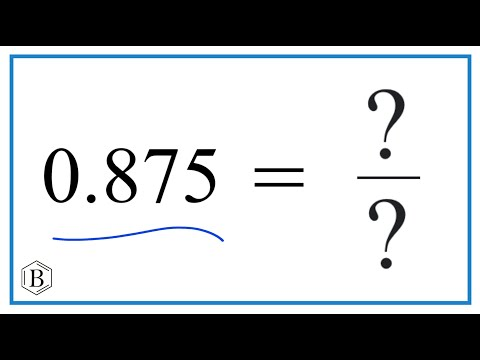Home » What is 0.875 as a Fraction? – Decimal to Fraction Conversion

# What is 0.875 as a Fraction? – Decimal to Fraction Conversion

What is 0.875 as a Fraction?

• A. 5/8
• B. 7/8
• C. 1/2
• D. 3/5
• E. 129/100

## Solution:

0.875 can be expressed as the fraction 7/8.

Here’s a quick explanation:

Since the decimal 0.875 is in the thousandths place, it can be expressed as a fraction over 1000. We, therefore, begin with 875/1000.

The greatest common divisor (GCD) of 875 and 1000, which is 125, can simplify this fraction. 7/8 is obtained by dividing the numerator (875), the denominator (1000), and 125.

So, therefore, 0.875 as a fraction is equal to 7/8.

## Understanding the Decimal Place

The first step is to understand the decimal place value to convert a decimal to a fraction. In this case, the number we have is 0.875. The “875” is in the thousandths place. In the decimal number system, the first digit to the right of the decimal point stands for tenths, the second for hundredths, and the third for thousandths. So, as a first step, the decimal 0.875 can be written as the fraction 875/1000.

## Simplifying the Fraction

Now, the next step is to simplify this fraction. The simplest form of a fraction is when the greatest common divisor (GCD) of the numerator and denominator is 1. This indicates that the numerator and denominator share no factors other than 1. 125 is the greatest common divisor of 875 and 1000 in the context of 875/1000.

## 0.875 as a Fraction in Simplest Form

To make 875/1000 easier to understand, we divide both the numerator (875) and the denominator (1000) by their greatest common divisor (GCD), which is 125. By doing this, we get 7/8 (because 875 ÷ 125 = 7 and 1000 ÷ 125 = 8). So, 0.875 can be written as 7/8, the easiest fraction to understand. This method can turn any decimal into its most basic fraction form.

In summary, to convert decimals to fractions, you need to understand the place values of decimals, write the decimal as a fraction, and then, if necessary, simplify that fraction.